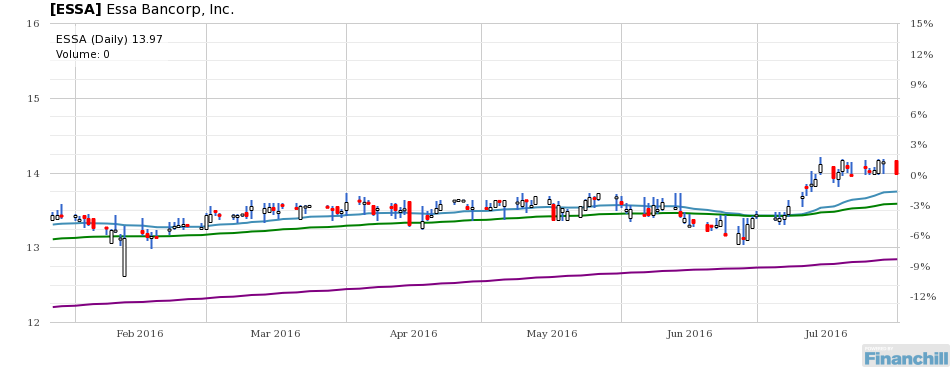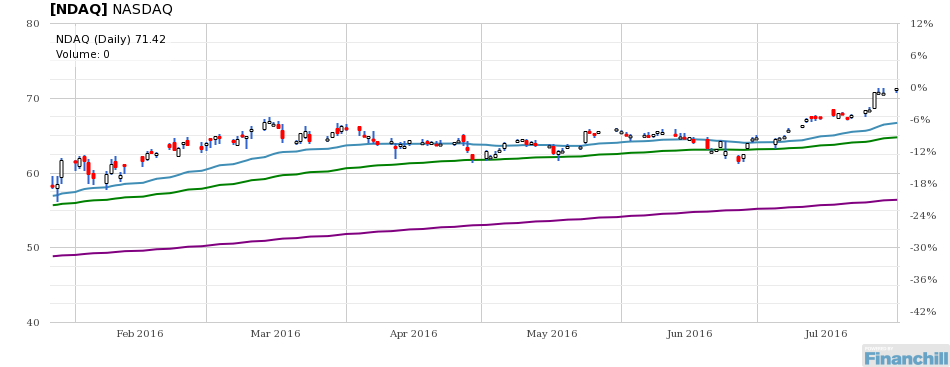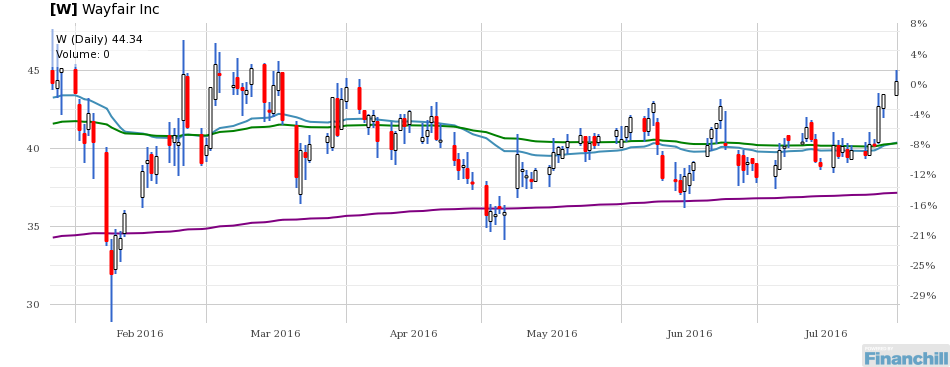# DIN Newsletter for August 2, 2016

## Stock Of The Day: Buy or Sell?

Risk levels may be somewhat lower than normal.

## CHARTS OF THE DAY

### Essa Bancorp, Inc. [ESSA]

Chart for ESSAESSA share price is \$13.97, which is 2% above its 21-day moving average of \$13.75.

ESSA share price is \$13.97, which is 3% above its 50-day moving average of \$13.59.

ESSA share price is \$13.97, which is 9% above its 200-day moving average of \$12.85.

### NASDAQ [NDAQ]

Chart for NDAQNDAQ share price is \$71.42, which is 7% above its 21-day moving average of \$66.71.

NDAQ share price is \$71.42, which is 10% above its 50-day moving average of \$64.77.

NDAQ share price is \$71.42, which is 27% above its 200-day moving average of \$56.42.

### Wayfair Inc [W]

Chart for WW share price is \$44.34, which is 10% above its 21-day moving average of \$40.38.

W share price is \$44.34, which is 10% above its 50-day moving average of \$40.33.

W share price is \$44.34, which is 19% above its 200-day moving average of \$37.16.

## Economic Indicators

### Total Market Cap / GDP

Chart for Total Market Cap / GDPThe ratio of the Total Market Capitalization (Wilshire 5000) to United States GDP is 1.2230. This is 41.1% above the historic median figure of 0.87.

The higher the Market Cap/GDP figure is relative to its median level, the higher the perceived risk in the market. Markets can remain overvalued for extended time periods.

### S&P Earnings Growth

Chart for S&P Earnings GrowthThe S&P Earnings Growth is -12.91. This is 213.6% below the historic median figure of 11.37.

When the S&P Earnings Growth trends lower, the stock market is perceived to have higher risk.

## Sentiment

### VIX

Chart for VIXThe VIX figure is 12.44. This is 29.9% below the historic median figure of 17.74.

The CBOE Volatility Index® (VIX®) is a key measure of market expectations of near-term volatility conveyed by S&P 500 stock index option prices. Historically, VIX levels above 50 correlate closely with periods of stock market panic while VIX levels below 20 are more typical during relatively calm periods in the market.